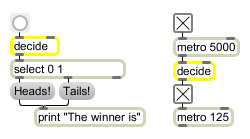# decide

Choose randomly between on and off (1 and 0)

## Description

decide outputs either a specific (reproducible) sequence of pseudo-random 1 s and 0 s or an unpredictable sequence of 0 s and 1 s depending on what "seed"-value is given.

## Arguments

Name Type Opt Description
seed int opt Sets a "seed" value to cause a specific (reproducible) sequence of pseudo-random 0 and 1 outputs to occur. If there is no argument, the time elapsed since system startup (an unpredictable value) is used as the seed, ensuring an unpredictable sequence of 0 and 1 outputs.

## Messages

 bang In left inlet: Causes a randomly chosen output of 1 or 0. int start [int] In left inlet: Same as bang. (inlet1) seed [int] In right inlet: A given "seed" number causes a specific (reproducible) sequence of pseudo-random 0 and 1 outputs to occur. The number 0 uses the time elapsed since system startup (an unpredictable value) as the seed, ensuring an unpredictable sequence of 0 and 1 outputs.

## Output

int: A 1 or a 0, chosen at random. With certain seed values, the output may seem at first to follow a "non-random" pattern, but over the course of many iterations the sequence becomes unpredictable and the balance between 1 and 0 becomes even.

## ExamplesSimulate a coin toss switch randomly between on and off